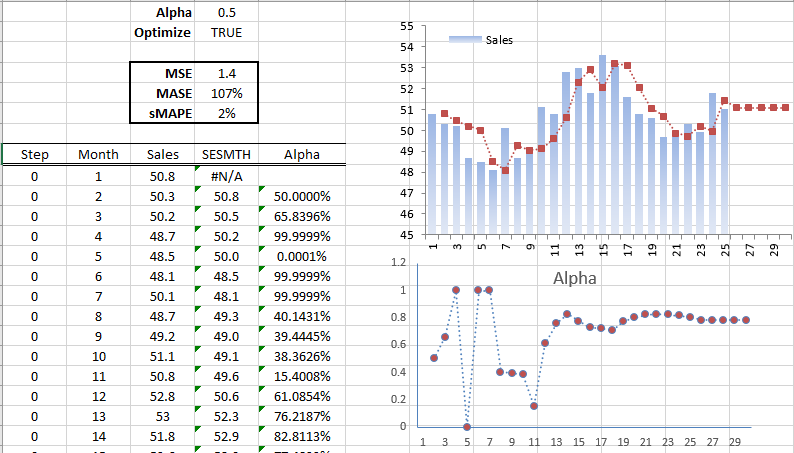# Brown's Simple Exponential Smoothing in Excel (SESMTH)

Check out our Simple (Brown) Exponential Smoothing tutorial below. The playlist contains tutorials that teach you how to utilize the optimization switch, calibrate with a training set, and calculate an in-sample forecast using NumXL.

Optimization on Calibration Dataset Optimization Off Forecasting

## Optimization On

In this video, we show you how to use Brown's simple exponential smoothing function in NumXL with an optimization switch for smoothing factors.

Video script

Scene 1:

Hello and welcome to the Exponential Smoothing Tutorial series. In this tutorial, we'll resume the demonstration of Brown simple exponential smoothing function and use the built-in optimizer in NumXL. For the sample data, we are using the same sales data of a hypothetical company.

Let's begin by selecting cell D9. Examine the cell formula in the formula toolbar, notice we already have a call for the simple exponential smoothing function so press F2 to edit.

Scene 2:

Click on the FX button found on the left side of the equation toolbar, this will invoke the function arguments dialog box for the simple exponential smoothing function.

Scene 3:

Notice that the cell in D2 is used for the optimize argument, so we don't need to change the formula just the value in D2 to turn on the optimizer. Now click cancel to go back to your worksheet.

Scene 4:

Select cell D2 and type in true or 1 then hit enter when done. Since we have the automatic calculation on, all of the values of the smooth time series are recalculated using optimal smoothing factor known as alpha. Notice the favorable changes in mean squared error, mean absolute scaled error, and symmetric mean absolute percentage error.

The calibrated simple exponential smoothing has a 10% lower mean absolute error than that of a naive reference model. Also, the function calculates the optimal value for alpha using the available data or information.

The available data increases with time, so the function calculates a new value each time. What about the alpha value in D1? Well, when the optimizer flag is turned on the function uses the past value of alpha as a starting value for the optimizer.

Scene 5:

Now let's examine the value of the smoothing parameter at each period. Select cell E10 and start typing in =SESMTH(. Then click on the FX button found on the left side of the equation toolbar, this will invoke the function arguments dialog box for the simple exponential smoothing function.

Scene 6:

Then specify the input cell range, this is the same cell range we used earlier for the forecast in column D. Lock the starting cell in the input range, enter a value of true or one in the order field, and for alpha let's use the same value in D1. Lock the cell by pressing F4.

For the optimized switch let's use the value in cell D2. Lock the cell by pressing F4 and then set the forecast time to zero or set to the value of the cell in A10. Lock the cell for column movement.

For return type, type in 1 for returning the value of the smoothing parameter, then click OK now.

Scene 7:

Then copy the formula to the cells below it. The value of the smoothing parameter at each period is calculated, now let's plot the values of the smoothing factor versus time.

Scene 8:

The graph shows the volatility of the computed alpha value throughout the sample in the first year. In the second year, the value of the smoothing factor is converging to around 0.8.

Does the simple exponential smoothing function use all of the values of alpha? Well, yes it does!

Can I force the simple exponential smoothing function to use one value for alpha? You could, but you would need to turn off the optimizer.

In a follow-up tutorial, we will calculate the value of alpha using a subset of the sample data then use the calibrated alpha for the rest of the sample data.Scene 9:

That's all for now, thank you for watching!